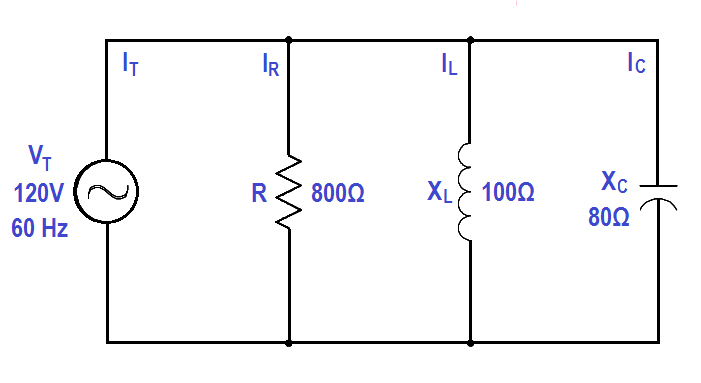# Calculate Power in Parallel RCL Circuit

An 800 Ω resistance, 100 Ω XL , and an 80 Ω XC are in parallel with a 120V, 60Hz source, as shown in Figure.Figure : Parallel R-C-L Circuit

Find:

1. Current, IT
2. Power Factor, pf
3. True Power, P
4. Reactive Power, Q
5. Apparent Power, S

Solution:

##### 1.Current, ITIT  =  0.34 amps

##### 2. Calculate Power factor, p.f.p.f. = cos (63.4º)

p.f. = 0.45

##### 4. Calculate True Power, P

P = EI cos θ

P = (120)(0.34)(0.45)

P = 18.36 watts

##### 5. Calculate Reactive Power, Q

Q = EI sin θ

Q = (120)(0.34)(0.89)

Q = 36.4 VAR

S = EI

S = (120)(0.34)

S = 40.8 VA